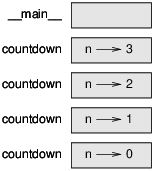# 5.9: Stack diagrams for recursive functions

$$\newcommand{\vecs}{\overset { \rightharpoonup} {\mathbf{#1}} }$$ $$\newcommand{\vecd}{\overset{-\!-\!\rightharpoonup}{\vphantom{a}\smash {#1}}}$$$$\newcommand{\id}{\mathrm{id}}$$ $$\newcommand{\Span}{\mathrm{span}}$$ $$\newcommand{\kernel}{\mathrm{null}\,}$$ $$\newcommand{\range}{\mathrm{range}\,}$$ $$\newcommand{\RealPart}{\mathrm{Re}}$$ $$\newcommand{\ImaginaryPart}{\mathrm{Im}}$$ $$\newcommand{\Argument}{\mathrm{Arg}}$$ $$\newcommand{\norm}{\| #1 \|}$$ $$\newcommand{\inner}{\langle #1, #2 \rangle}$$ $$\newcommand{\Span}{\mathrm{span}}$$ $$\newcommand{\id}{\mathrm{id}}$$ $$\newcommand{\Span}{\mathrm{span}}$$ $$\newcommand{\kernel}{\mathrm{null}\,}$$ $$\newcommand{\range}{\mathrm{range}\,}$$ $$\newcommand{\RealPart}{\mathrm{Re}}$$ $$\newcommand{\ImaginaryPart}{\mathrm{Im}}$$ $$\newcommand{\Argument}{\mathrm{Arg}}$$ $$\newcommand{\norm}{\| #1 \|}$$ $$\newcommand{\inner}{\langle #1, #2 \rangle}$$ $$\newcommand{\Span}{\mathrm{span}}$$$$\newcommand{\AA}{\unicode[.8,0]{x212B}}$$

In Section 3.9, we used a stack diagram to represent the state of a program during a function call. The same kind of diagram can help interpret a recursive function.

Every time a function gets called, Python creates a frame to contain the function’s local variables and parameters. For a recursive function, there might be more than one frame on the stack at the same time.

Figure $$\PageIndex{1}$$ shows a stack diagram for countdown called with n = 3.Figure $$\PageIndex{1}$$: Stack diagram.

As usual, the top of the stack is the frame for __main__. It is empty because we did not create any variables in __main__ or pass any arguments to it.

The four countdown frames have different values for the parameter n. The bottom of the stack, where n=0, is called the base case. It does not make a recursive call, so there are no more frames.

As an exercise, draw a stack diagram for print_n called with s = 'Hello' and n=2. Then write a function called do_n that takes a function object and a number, n, as arguments, and that calls the given function n times.

This page titled 5.9: Stack diagrams for recursive functions is shared under a CC BY-NC 3.0 license and was authored, remixed, and/or curated by Allen B. Downey (Green Tea Press) via source content that was edited to the style and standards of the LibreTexts platform; a detailed edit history is available upon request.